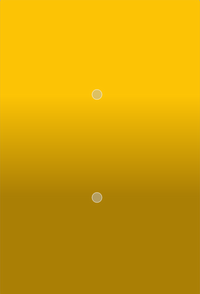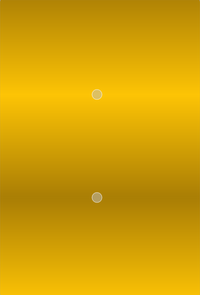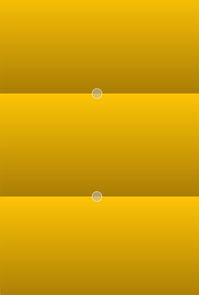The QLinearGradient class is used in combination with QBrush to specify a linear gradient brush. More...

 Header: #include qmake: QT += gui Inherits: QGradient

## Public Functions

 QLinearGradient() QLinearGradient(const QPointF &start, const QPointF &finalStop) QLinearGradient(qreal x1, qreal y1, qreal x2, qreal y2) QPointF finalStop() const void setFinalStop(const QPointF &stop) void setFinalStop(qreal x, qreal y) void setStart(const QPointF &start) void setStart(qreal x, qreal y) QPointF start() const
• 10 public functions inherited from QGradient

## Detailed Description

The QLinearGradient class is used in combination with QBrush to specify a linear gradient brush.

Linear gradients interpolate colors between start and end points. Outside these points the gradient is either padded, reflected or repeated depending on the currently set spread method:PadSpread (default) ReflectSpread RepeatSpread

The colors in a gradient is defined using stop points of the QGradientStop type, i.e. a position and a color. Use the QGradient::setColorAt() or the QGradient::setStops() function to define the stop points. It is the gradient's complete set of stop points that describes how the gradient area should be filled. If no stop points have been specified, a gradient of black at 0 to white at 1 is used.

In addition to the functions inherited from QGradient, the QLinearGradient class provides the finalStop() function which returns the final stop point of the gradient, and the start() function returning the start point of the gradient.

## Member Function Documentation

Constructs a default linear gradient with interpolation area between (0, 0) and (1, 1).

### QLinearGradient::QLinearGradient(const QPointF &start, const QPointF &finalStop)

Constructs a linear gradient with interpolation area between the given start point and finalStop.

Note: The expected parameter values are in pixels.

Constructs a linear gradient with interpolation area between (x1, y1) and (x2, y2).

Note: The expected parameter values are in pixels.

### QPointF QLinearGradient::finalStop() const

Returns the final stop point of this linear gradient in logical coordinates.

### void QLinearGradient::setFinalStop(const QPointF &stop)

Sets the final stop point of this linear gradient in logical coordinates to stop.

This function was introduced in Qt 4.2.

### void QLinearGradient::setFinalStop(qrealx, qrealy)

This is an overloaded function.

Sets the final stop point of this linear gradient in logical coordinates to x, y.

This function was introduced in Qt 4.2.

### void QLinearGradient::setStart(const QPointF &start)

Sets the start point of this linear gradient in logical coordinates to start.

This function was introduced in Qt 4.2.

### void QLinearGradient::setStart(qrealx, qrealy)

This is an overloaded function.

Sets the start point of this linear gradient in logical coordinates to x, y.

This function was introduced in Qt 4.2.